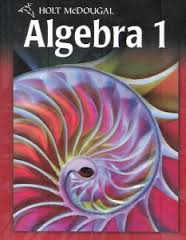# Lap 4 Quiz 1

30 Questions | Total Attempts: 870SettingsQuiz covering 4-1 Identifying Linear Functions through 4-5 Direct Variation

• 1.
Which of the following functions is NOT a linear function?
• A.
• B.
• C.
• D.
• 2.
What is the x-intercept of  ?
• A.

2

• B.

3

• C.

6

• D.

Not here

• 3.
Which is the graph of the line described by the equation ?
• A.
• B.
• C.
• D.

Not here

• 4.
Which of the following set of ordered pairs satisfy a linear function?
• A.

(–2, 5), (–1, 2), (0, 1), (1, 2), (2, 5)

• B.

(–4, 1), (–2, 2), (0, 4), (2, 6), (4, 8)

• C.

(2, 5), (4, 1), (6, –3), (8, –7), (10, –11)

• D.

(–2, 2), (–1, 1), (0, 0), (1, 1), (2, 2)

• 5.
At a fair, hamburgers sell for \$3.00 each and hot dogs sell for \$1.50 each. The equation 3x + 1.5y = 30 describes the number of hamburgers and hot dogs a family can buy with \$30.  What does the y-intercept represent?
• A.

The number of hamburgers they can buy if they buy no hot dogs

• B.

The number of hot dogs they can buy if they buy no hamburgers

• C.

The cost of x number of hamburgers

• D.

The cost of y number of hot dogs

• 6.
What is the slope of a vertical line?
• A.

0

• B.

Undefined

• C.

Positive

• D.

Negative

• 7.
The table shows the distance traveled at different times during a car trip. During which time period is the rate of change the greatest?
• A.

Between 0 and 1 hour

• B.

Between 1 and 3 hours

• C.

Between 3 and 4 hours

• D.

Between 4 and 6 hours

• 8.
What is the slope of a horizontal line?
• A.

0

• B.

Undefined

• C.

Positive

• D.

Negative

• 9.
What is the slope of a line that moves down and to the left?
• A.

0

• B.

Undefined

• C.

Positive

• D.

Negative

• 10.
What is the y-intercept of  ?
• A.

2

• B.

3

• C.

6

• D.

Not here

• 11.
At a fair, hamburgers sell for \$3.00 each and hot dogs sell for \$1.50 each. The equation 3x + 1.5y = 30 describes the number of hamburgers and hot dogs a family can buy with \$30.  What does the x-intercept represent?
• A.

The number of hamburgers they can buy if they buy no hot dogs

• B.

The number of hot dogs they can buy if they buy no hamburgers

• C.

The cost of x number of hamburgers

• D.

The cost of y number of hot dogs

• 12.
What is the slope of a line that moves up and to the left?
• A.

0

• B.

Undefined

• C.

Positive

• D.

Negative

• 13.
What is the x-intercept of  ?
• A.

−2

• B.

4

• C.

8

• D.

Not here

• 14.
What is the slope of a line that moves up and to the right?
• A.

0

• B.

Undefined

• C.

Positive

• D.

Negative

• 15.
What is the slope of a line that moves down and to the right?
• A.

0

• B.

Undefined

• C.

Positive

• D.

Negative

• 16.
Which is the graph of the line described by the equation ?
• A.
• B.
• C.
• D.

Not here

• 17.
Which line has a slope of ?
• A.
• B.
• C.
• D.
• 18.
Which line has a slope of ?
• A.
• B.
• C.
• D.
• 19.
Which line has a slope of 2?
• A.
• B.
• C.
• D.
• 20.
Which line has a slope of ?
• A.
• B.
• C.
• D.
• 21.
Find the slope of the line.  Then tell what the slope represents.
• A.

Slope = 1; water in tank is rising at a rate of 1 foot per minute

• B.

Slope = −10; water in tank is dropping at a rate of 10 feet per minute

• C.

Slope = −1; water in tank is dropping at a rate of 1 foot per minute

• D.

Slope = 10; water in tank is dropping at a rate of 10 feet every 10 minutes

• 22.
Find the slope of the line that contains the points (−3, 4) and (5, 2).
• A.
• B.
• C.
• D.

Not here

• 23.
Find the slope of the line that contains the points (−3, 4) and (2, 2).
• A.
• B.
• C.
• D.

Not here

• 24.
Find the slope of the line that contains the points (−3, −5) and (1, 3).
• A.
• B.
• C.
• D.

Not here

• 25.
What is the slope of the line that passes through (−2, 9) and (4, −3)
• A.

2

• B.

4

• C.

6

• D.

Not here

Related TopicsBack to top